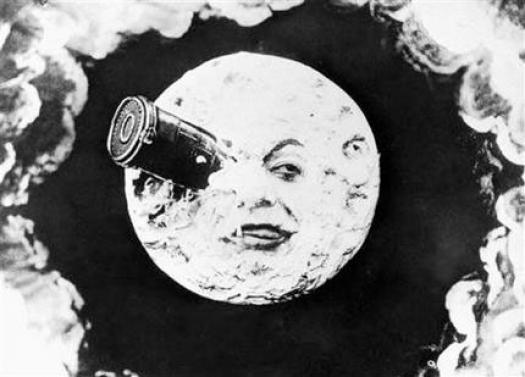# Moon Practice Quiz

15 Questions | Total Attempts: 1086Settings• 1.
Why does the moon move through the sky?
• A.

Because it is revolving around the Earth

• B.

Because of the Earth's rotation

• C.

Because of the sun's rotation

• D.

Because the moon is moving away from the Earth

• 2.
What is the moon phase shown below
• A.

New Moon

• B.

First Quarter

• C.

Third Quarter

• D.

Full Moon

• 3.
Which letter represents a new moon (make sure to look at the direction that the sun is coming from).
• A.

T

• B.

R

• C.

S

• D.

Q

• 4.
Which letter represents a first quarter moon (make sure to look at the direction that the sun is coming from)?
• A.

T

• B.

R

• C.

S

• D.

Q

• 5.
Which letter represents a third quarter moon (make sure to look at the direction that the sun is coming from)?
• A.

T

• B.

R

• C.

S

• D.

Q

• 6.
What is the order of the objects for a solar eclipse?
• A.

Sun, Moon, Earth

• B.

Earth, Sun, Moon

• C.

Sun, Earth, Moon

• D.

Earth, Moon, Mars

• 7.
What is the order of the objects for a lunar eclipse?
• A.

Sun, Moon, Earth

• B.

Earth, Sun, Moon

• C.

Sun, Earth, Moon

• D.

Earth, Sun, Mars

• 8.
A solar eclipse is when the
• A.

Shadow of Mars falls on the Earth

• B.

Shadow of the sun falls on the moon

• C.

Shadow of the moon falls on the Earth

• D.

Shadow of the Earth falls on the Moon

• 9.
A lunar eclipse is when the
• A.

Shadow of Mars falls on the Earth

• B.

Shadow of the sun falls on the moon

• C.

Shadow of the moon falls on the Earth

• D.

Shadow of the Earth falls on the Moon

• 10.
What is this an image of?
• A.

A black hole

• B.

A lunar eclipse

• C.

A solar eclipse

• D.

A terra eclipse

• 11.
What is this a picture of?
• A.

A harvest moon

• B.

A lunar eclipse

• C.

A solar eclipse

• D.

A new moon

• 12.
What are tidal changes caused by?
• A.

The moon revolving around the Earth

• B.

Wind patterns caused by the moon

• C.

Ocean currents

• D.

The Earth revolving around the sun

• 13.
We have tides mostly because of
• A.

The sun's gravitational pull

• B.

The moon's gravitational pull

• C.

The uneven heating of the Earth

• D.

Winds

• 14.
How many high tides are there a day?
• A.

1

• B.

2

• C.

3

• D.

4

• 15.
Do tides come at the same time each day?
• A.

Yes

• B.

No, they come every other day

• C.

No, and you can't predict them

• D.

No, but you can predict them

Related TopicsBack to top